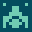# recognizers

#### A collection of recognizers

```\
\ Purpose: Number recognizers based on Forth CORE (only?)
\
\ Author: Matthias Trute
\ Date: Oct 10, 2016
\
\ not really smart code, tested with old gforth's and vfxlin's

decimal

' noop :NONAME POSTPONE LITERAL ; DUP  recognizer: r:num
' noop :NONAME POSTPONE 2LITERAL ; DUP recognizer: r:dnum

\ helper words for number recognizers

\ set BASE according the the character at addr
\ return string is stripped of it if found.

create num-bases 10 , 16 , 2 , 8 ,
over c@ 35 - dup 0 4 within if
cells num-bases + @ base ! 1 /string
else
drop
then
;

\ check for - sign. return string is
\ without it if found.

over c@ [char] - = dup >r
if 1 /string then r>
;
\ check for the 'c' syntax for single
\ characters. Assumes 1 char = 1

: rec:char ( addr len -- n r:num | r:fail )
3 = if \ a three character string
dup c@ [char] ' = if \ starts with a '
dup 2 + c@ [char] ' = if \ and ends with a '
1+ c@ r:num exit
then
then
then
drop r:fail
;

\ check for double number input
\ strictly following Forth 2012 spec
\ that is <base-prefix><sign><digits><dot>
\ e.g. #-42. but not -#42. or -#4.2
\ this recognizer is very strict about it.

decimal

: rec:dnum ( addr len -- d r:dnum | rfail )
\ simple syntax check: last character in addr/len is a dot . ?
2dup + 1- c@ [char] . = if
1-                  \ strip trailing dot
base @ >r set-base  \ save BASE and check'n'set for a new one.
-sign? >r           \ check for the minus sign character
2>r 0 0 2r> >number \ do the actual conversion
swap if 2drop 2drop r:fail else nip if dnegate then r:dnum then
else
2drop r:fail  \ no, it cannot be a double cell number.
then
;

\ check for single cell number input

\ number format according to Forth 2012
\ <prefix><sign><digits>
\ #-42, but not -#42
\

: rec:snum ( addr len -- n r:num | rfail )
base @ >r \ save BASE
set-base -sign? >r
2>r 0 0 2r> >number ( -- d addr' len' )
r> r> base ! >r \ restore BASE
\ a number and only a number?
nip if 2drop r> drop r:fail exit then ( -- d )
\ a single cell number?
if r> drop r:fail else r> if negate then r:num then
;

\ combine them, just for testing purposes
3 RECOGNIZER VALUE recstack:numbers

' rec:char ' rec:dnum ' rec:snum 3 recstack:numbers SET-RECOGNIZERS

: REC:NUM ( addr len -- n R:NUM | d R:DNUM | R:FAIL )
recstack:numbers DO-RECOGNIZER
;

\
\ ------------- Unit tests for all of the above ----------
\

VERBOSE ON

T{ S" 'z'"  rec:char -> char z r:num }T
T{ S" 'z '" rec:char -> r:fail }T

T{ S" 1234."  rec:dnum -> 1234. r:dnum }T
T{ S" -1234." rec:dnum -> -1234. r:dnum }T
T{ S" \$1234." rec:dnum -> \$1234. r:dnum }T
T{ S" \$-1234." rec:dnum -> \$-1234. r:dnum }T

T{ S" -\$1234." rec:dnum -> r:fail }T
T{ S" 1234"   rec:dnum -> r:fail }T
T{ S" ABCXYZ" rec:dnum -> r:fail }T

T{ S" 1234"   rec:snum ->  1234  r:num }T
T{ S" -1234"  rec:snum -> -1234  r:num }T
T{ S" \$1234"  rec:snum -> \$1234  r:num }T
T{ S" \$-1234" rec:snum -> \$-1234 r:num }T
T{ S" #1234"  rec:snum ->  1234  r:num }T
T{ S" %1110"  rec:snum -> %1110  r:num }T

T{ S" #123a"  rec:snum -> r:fail }T
T{ S" -\$1234" rec:snum -> r:fail }T
T{ S" 1234."  rec:snum -> r:fail }T
T{ S" ABCXYZ" rec:snum -> r:fail }T

```

## by mtrute